# Problem: In each of the parts of this question, a nucleus undergoes a nuclear decay. Determine the resulting nucleus in each case.A.  undergoes alpha decay. Determine the resulting nucleus.B.  undergoes beta-minus decay. Determine the resulting nucleus.C.  undergoes beta-plus decay. Determine the resulting nucleus.D.  undergoes gamma decay. Determine the resulting nucleus.

🤓 Based on our data, we think this question is relevant for Professor Massa's class at HUNTER.

###### FREE Expert Solution

In most nuclear reaction the number of protons in an element is affected and so the identity of the element changes.

A. An alpha decay or alpha emission occurs when an alpha particle is ejected to create a new element.  An alpha particle is comprised of 2 protons and 2 neutrons.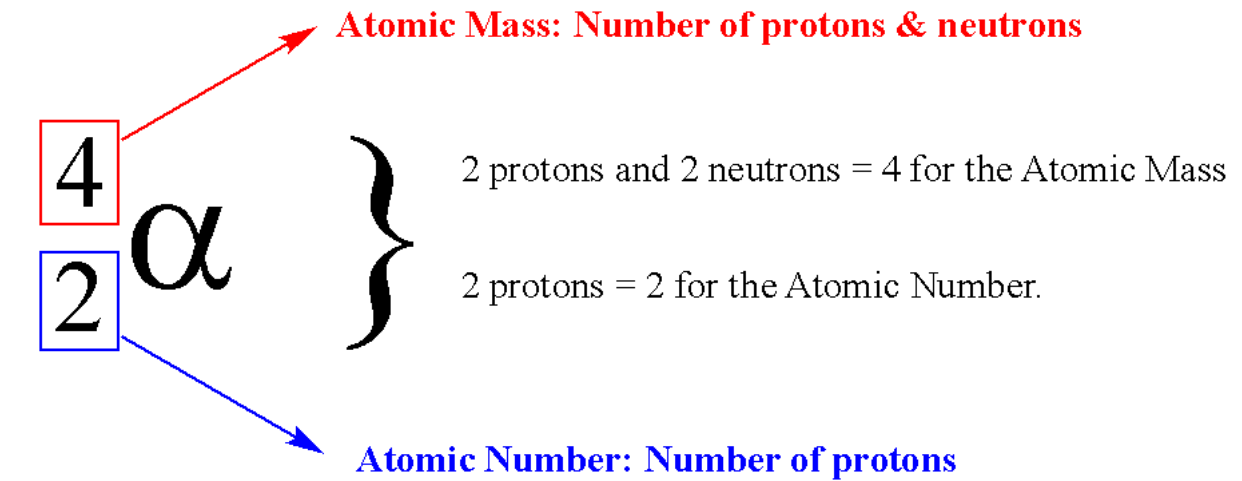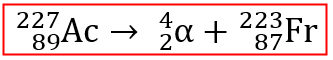The resulting nucleus is 223Fr###### Problem Details

In each of the parts of this question, a nucleus undergoes a nuclear decay. Determine the resulting nucleus in each case.

A.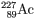undergoes alpha decay. Determine the resulting nucleus.

B.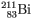undergoes beta-minus decay. Determine the resulting nucleus.

C.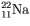undergoes beta-plus decay. Determine the resulting nucleus.

D.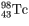undergoes gamma decay. Determine the resulting nucleus.